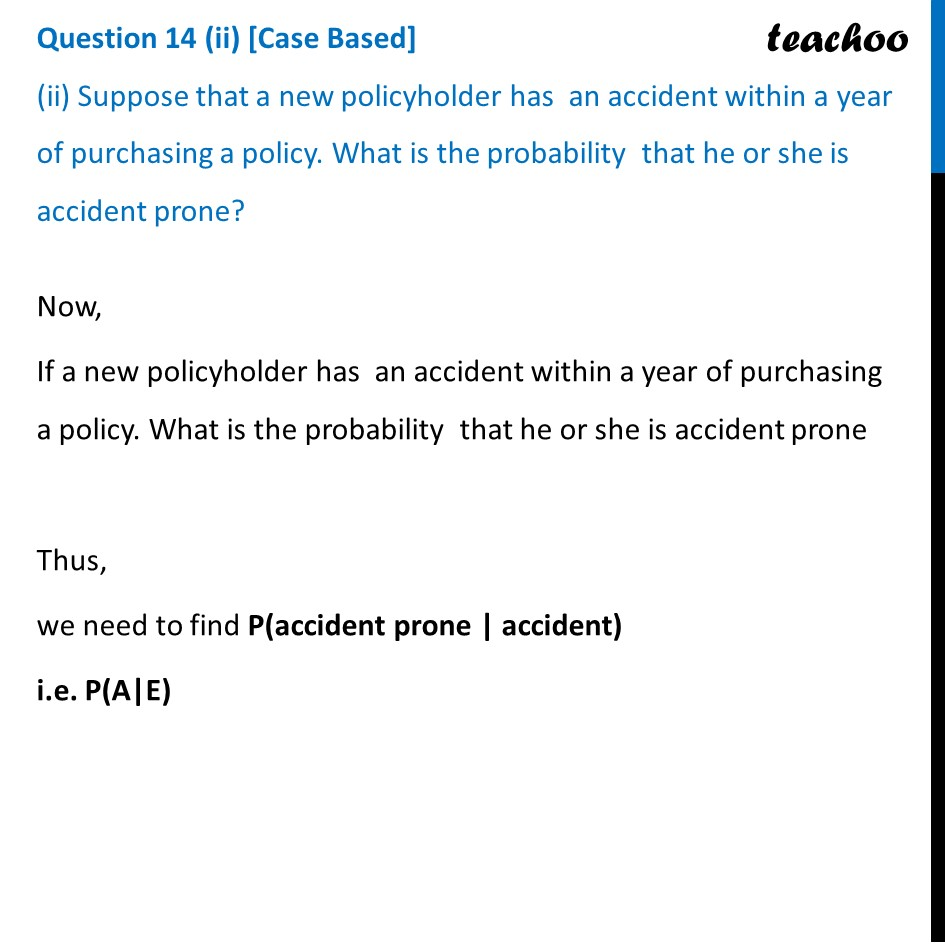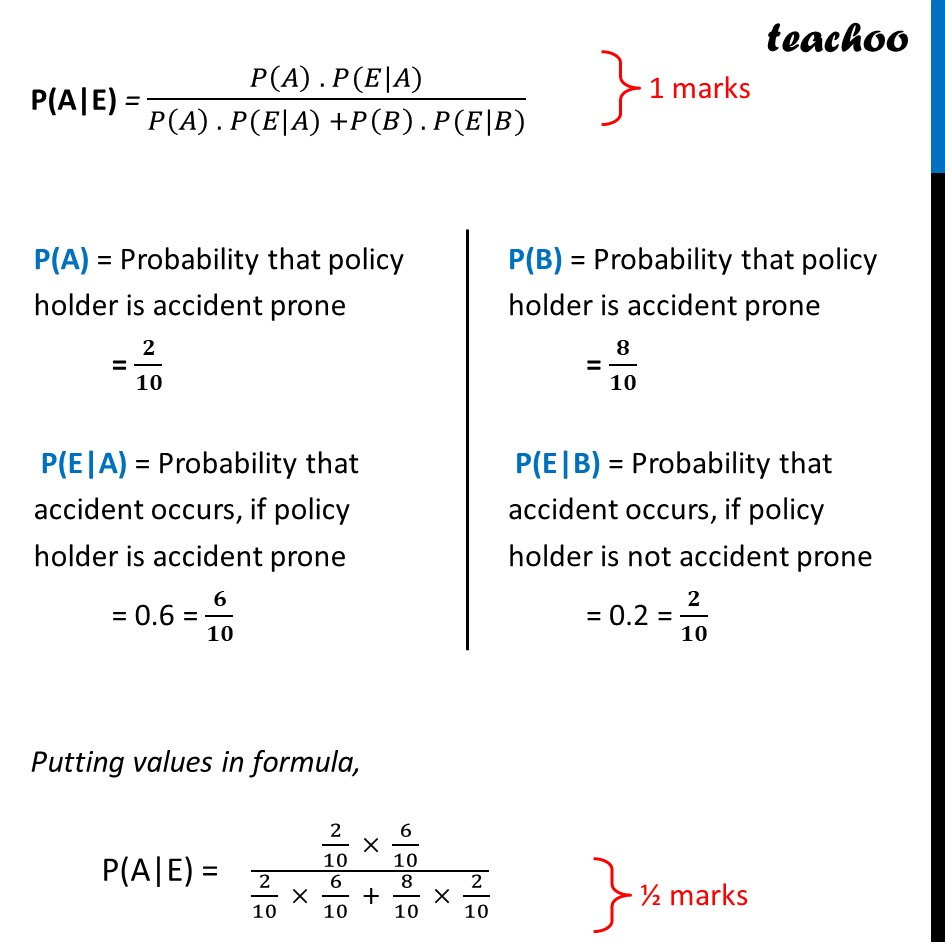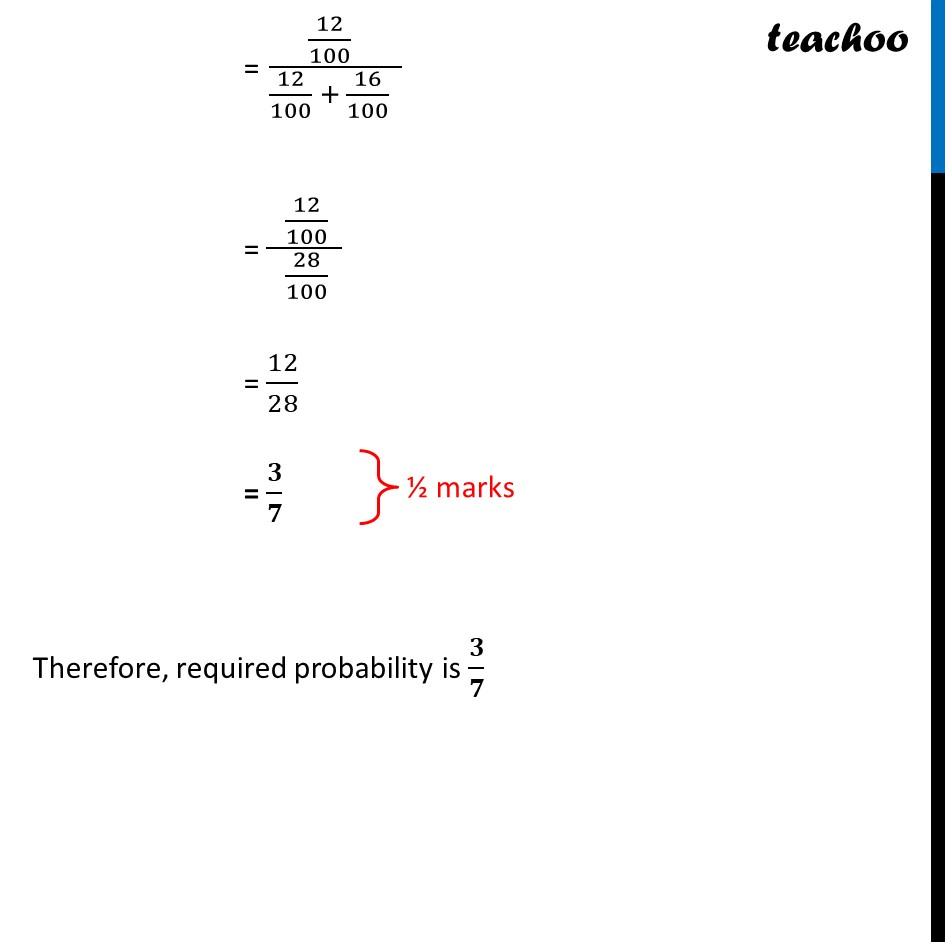CBSE Class 12 Sample Paper for 2022 Boards (For Term 2)

Class 12
Solutions of Sample Papers and Past Year Papers - for Class 12 Boards

##Learn in your speed, with individual attention - Teachoo Maths 1-on-1 Class

### Transcript

An insurance company believes that people can be divided into two classes: those who are accident prone and those who are not. The company’s statistics show that an accident-prone person will have an accident at sometime within a fixed one-year period with probability 0.6, whereas this probability is 0.2 for a person who is not accident prone. The company knows that 20 percent of the population is accident prone. Based on the given information, answer the following questions Based on the given information, answer the following questions. (i) what is the probability that a new policyholder will have an accident within a year of purchasing a policy? (ii) Suppose that a new policyholder has an accident within a year of purchasing a policy. What is the probability that he or she is accident prone? Let A : policy holder who is accident prone B : policy holder who is not accident prone And, E: Probability person will have an accident within 1 year of purchasing policy Given P(A) = 20% = 𝟐/𝟏𝟎 P(B) = 80% = 𝟖/𝟏𝟎 And, Probability accident-prone person will have an accident at sometime within a fixed one-year period with probability 0.6, P(E|A) = 0.6 Also, Probability not accident-prone person will have an accident at sometime within a fixed one-year period is 0.2, P(E|B) = 0.2 Question 14 (i) [Case Based] (i) what is the probability that a new policyholder will have an accident within a year of purchasing a policy? We need to find what is the probability that a new policyholder will have an accident within a year of purchasing a policy P(new holder will have an accident) = P(policy holder is accident prone) × P(person will have accident if he is accident prone) + P(policy holder is not accident prone) × P(person will have accident if he is accident prone) = P(A) × P(E|A) + P(B) × P(E|B) = 2/10 × 0.6+8/10 × 0.2 = 2/10 ×6/10+8/10 ×2/10 = (12 + 16)/100 = 28/100 = 0.28 Question 14 (ii) [Case Based] (ii) Suppose that a new policyholder has an accident within a year of purchasing a policy. What is the probability that he or she is accident prone? Now, If a new policyholder has an accident within a year of purchasing a policy. What is the probability that he or she is accident prone Thus, we need to find P(accident prone | accident) i.e. P(A|E) P(A|E) = (𝑃(𝐴) . 𝑃(𝐸|𝐴))/(𝑃(𝐴) . 𝑃(𝐸|𝐴) +𝑃(𝐵) . 𝑃(𝐸|𝐵)) "P(A)" = Probability that policy holder is accident prone = 𝟐/𝟏𝟎 "P(E|A)" = Probability that accident occurs, if policy holder is accident prone = 0.6 = 𝟔/𝟏𝟎 "P(B)" = Probability that policy holder is accident prone = 𝟖/𝟏𝟎 "P(E|B)" = Probability that accident occurs, if policy holder is not accident prone = 0.2 = 𝟐/𝟏𝟎 Putting values in formula, P(A|E) = (2/10 × 6/10)/(2/10 × 6/10 + 8/10 × 2/10) = (12/100 )/(12/100 + 16/100 ) = ( 12/100 )/( 28/100 ) = 12/28 = 𝟑/𝟕 Therefore, required probability is 𝟑/𝟕